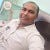# Strong Number in C Programming

#include <stdio.h>
#include <stdlib.h>
int main()
{
int i, sum=0,fact,n,num,rem;
printf(“Enter a Number\n”);
scanf(“%d”,&n);
num=n;
while(n>0)
{
rem=n%10;
fact=1;
for(i=1;i<=rem;i++)
{
fact=fact*i;
}
sum=sum+fact;
n=n/10;
}
if(num==sum)
{
printf(“%d is a Strong Number”,num);
}
else
{
printf(“%d is not a Strong Number”,num);
}
return 0;
}

For a detailed explanation,

Strong Number in C

# C Program to Find LCM of Two Numbers

#include<stdio.h>
int main()
{
int n1,n2,greater,smaller,i=1,lcm;
printf(“Enter two numbers\n”);
scanf(“%d%d”,&n1,&n2);
if(n1>n2)
{
greater=n1;
smaller=n2;
}
else
{
greater=n2;
smaller=n1;
}
while(1)
{
lcm = greater * i;
if((lcm%smaller)==0)
{
printf(“LCM of %d and %d is %d”, n1,n2,lcm);
break;
}
i++;
}
return 0;
}

# Output

For a detailed explanation with the logic

# Interview Questions On C

In this article, you can find more useful interview questions on c programming. If you master all these questions, I can guarantee you that you will definitely get success in all type of c programming interviews.

So there are thousands of interview questions on c programming. It is not possible…